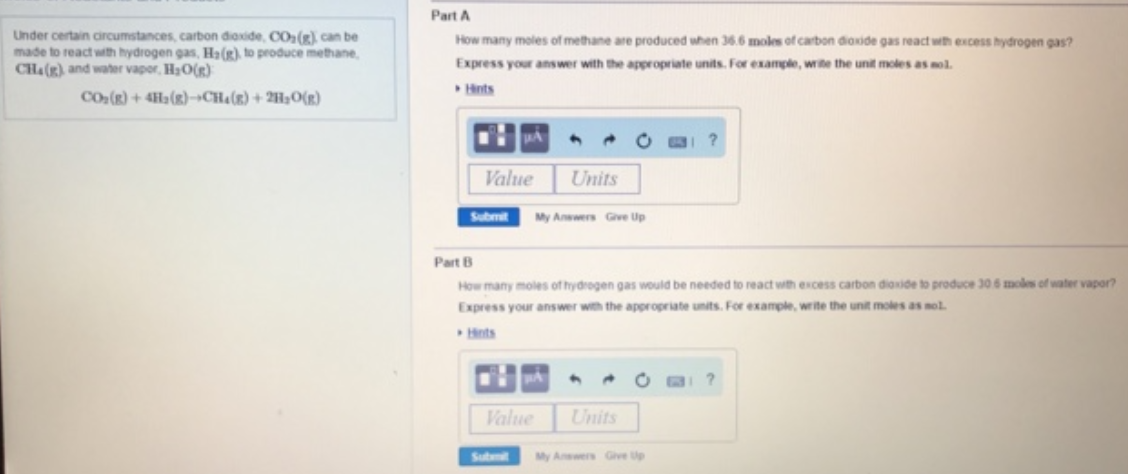# Problem: Under certain circumstances, carbon dioxide, CO2 (g) can be made to react with hydrogen gas, H2 (g) to produce methane, CH4 (g) and water vapour H2O (g).CO2 (g) + 4H2 (g) → CH4 (g) + 2H2O (g) Part AHow many moles of methane are produced when 36.6 moles of carbon dioxide gas react with excess hydrogen gas.Part BHow many moles of hydrogen gas would be needed to react with excess carbon dioxide to produce 30.6 moles of water.Express ye answer with the appropriate units.

###### FREE Expert Solution
100% (275 ratings)###### Problem Details

Under certain circumstances, carbon dioxide, CO(g) can be made to react with hydrogen gas, H2 (g) to produce methane, CH4 (g) and water vapour H2O (g).

CO2 (g) + 4H2 (g) → CH4 (g) + 2H2O (g)

Part A

How many moles of methane are produced when 36.6 moles of carbon dioxide gas react with excess hydrogen gas.

Part B

How many moles of hydrogen gas would be needed to react with excess carbon dioxide to produce 30.6 moles of water.

Express ye answer with the appropriate units.Frequently Asked Questions

What scientific concept do you need to know in order to solve this problem?

Our tutors have indicated that to solve this problem you will need to apply the Stoichiometry concept. You can view video lessons to learn Stoichiometry. Or if you need more Stoichiometry practice, you can also practice Stoichiometry practice problems.

What professor is this problem relevant for?

Based on our data, we think this problem is relevant for Professor Nikobakht's class at Montgomery County Community College.